# tidyterraThe goal of {tidyterra} is to provide common methods of the tidyverse packages for objects created with the {terra} package: SpatRaster and SpatVector. It also provides geoms for plotting these objects with {ggplot2}.

## Overview

{tidyverse} methods implemented on {tidyterra} works differently depending on the type of Spat* object:

• SpatVector: the methods are implemented using `terra::as.data.frame()` coercion. Rows correspond to geometries and columns correspond to attributes of the geometry.

• SpatRaster: The implementation on SpatRaster objects differs, since the methods could be applied to layers or to cells. {tidyterra} overall approach is to treat the layers as columns of a tibble and the cells as rows (i.e. `select(SpatRaster, 1)` would select the first layer of a SpatRaster).

The methods implemented return the same type of object used as input, unless the expected behavior of the method is to return another type of object, (for example, `as_tibble()` would return a tibble).

Current methods and functions provided by {tidyterra} are:

tidyverse method SpatVector SpatRaster
`tibble::as_tibble()` ✔️ ✔️
`dplyr::select()` ✔️ ✔️ Select layers
`dplyr::mutate()` ✔️ ✔️ Create /modify layers
`dplyr::transmute()` ✔️ ✔️
`dplyr::filter()` ✔️ ✔️ Modify cells values and (additionally) remove outer cells.
`dplyr::slice()` ✔️ ✔️ Additional methods for slicing by row and column.
`dplyr::pull()` ✔️ ✔️
`dplyr::rename()` ✔️ ✔️
`dplyr::relocate()` ✔️ ✔️
`dplyr::distinct()` ✔️
`dplyr::arrange()` ✔️
`dplyr::glimpse()` ✔️ ✔️
`dplyr::inner_join()` family ✔️
`dplyr::summarise()` ✔️
`dplyr::group_by()` family ✔️
`dplyr::rowwise()` ✔️
`dplyr::count()`, `tally()` ✔️
`dplyr::bind_cols()` / `dplyr::bind_rows()` ✔️ as `bind_spat_cols()` / `bind_spat_rows()`
`tidyr::drop_na()` ✔️ ✔️ Remove cell values with `NA` on any layer. Additionally, outer cells with `NA` are removed.
`tidyr::replace_na()` ✔️ ✔️
`ggplot2::autoplot()` ✔️ ✔️
`ggplot2::fortify()` ✔️ to sf via `sf::st_as_sf()` To a tibble with coordinates.
`ggplot2::geom_*()` ✔️ `geom_spatvector()` ✔️ `geom_spatraster()` and `geom_spatraster_rgb()`.

## :exclamation: A note on performance

{tidyterra} is conceived as a user-friendly wrapper of {terra} using the {tidyverse} methods and verbs. This approach therefore has a cost in terms of performance.

If you are a heavy user of {terra} or you need to work with big raster files, {terra} is much more focused on terms of performance. When possible, each function of {tidyterra} references to its equivalent on {terra}.

As a rule of thumb if your raster has less than 10.000.000 data slots counting cells and layers (i.e. `terra::ncell(your_rast)*terra::nlyr(your_rast) < 10e6`) you are good to go with {tidyterra}.

When plotting rasters, resampling is performed automatically (as `terra::plot()` does, see the help page). You can adjust this with the `maxcell` parameter.

## Installation

Install {tidyterra} from CRAN:

``install.packages("tidyterra")``

You can install the development version of {tidyterra} like so:

``remotes::install_github("dieghernan/tidyterra")``

Alternatively, you can install {tidyterra} using the r-universe:

``````# Enable this universe
options(repos = c(
dieghernan = "https://dieghernan.r-universe.dev",
CRAN = "https://cloud.r-project.org"
))
install.packages("tidyterra")``````

## Example

### SpatRasters

This is a basic example which shows you how to manipulate and plot SpatRaster objects:

``````library(tidyterra)
library(terra)

# Temperatures
rastertemp <- rast(system.file("extdata/cyl_temp.tif", package = "tidyterra"))

rastertemp
#> class       : SpatRaster
#> dimensions  : 87, 118, 3  (nrow, ncol, nlyr)
#> resolution  : 3881.255, 3881.255  (x, y)
#> extent      : -612335.4, -154347.3, 4283018, 4620687  (xmin, xmax, ymin, ymax)
#> coord. ref. : World_Robinson
#> source      : cyl_temp.tif
#> names       :   tavg_04,   tavg_05,  tavg_06
#> min values  :  1.885463,  5.817587, 10.46338
#> max values  : 13.283829, 16.740898, 21.11378

# Rename
rastertemp <- rastertemp %>%
rename(April = tavg_04, May = tavg_05, June = tavg_06)

# Facet all layers
library(ggplot2)

ggplot() +
geom_spatraster(data = rastertemp) +
facet_wrap(~lyr, ncol = 2) +
scale_fill_whitebox_c(
palette = "muted",
labels = scales::label_number(suffix = "º"),
n.breaks = 12,
guide = guide_legend(reverse = TRUE)
) +
labs(
fill = "",
title = "Average temperature in Castille and Leon (Spain)",
subtitle = "Months of April, May and June"
)``````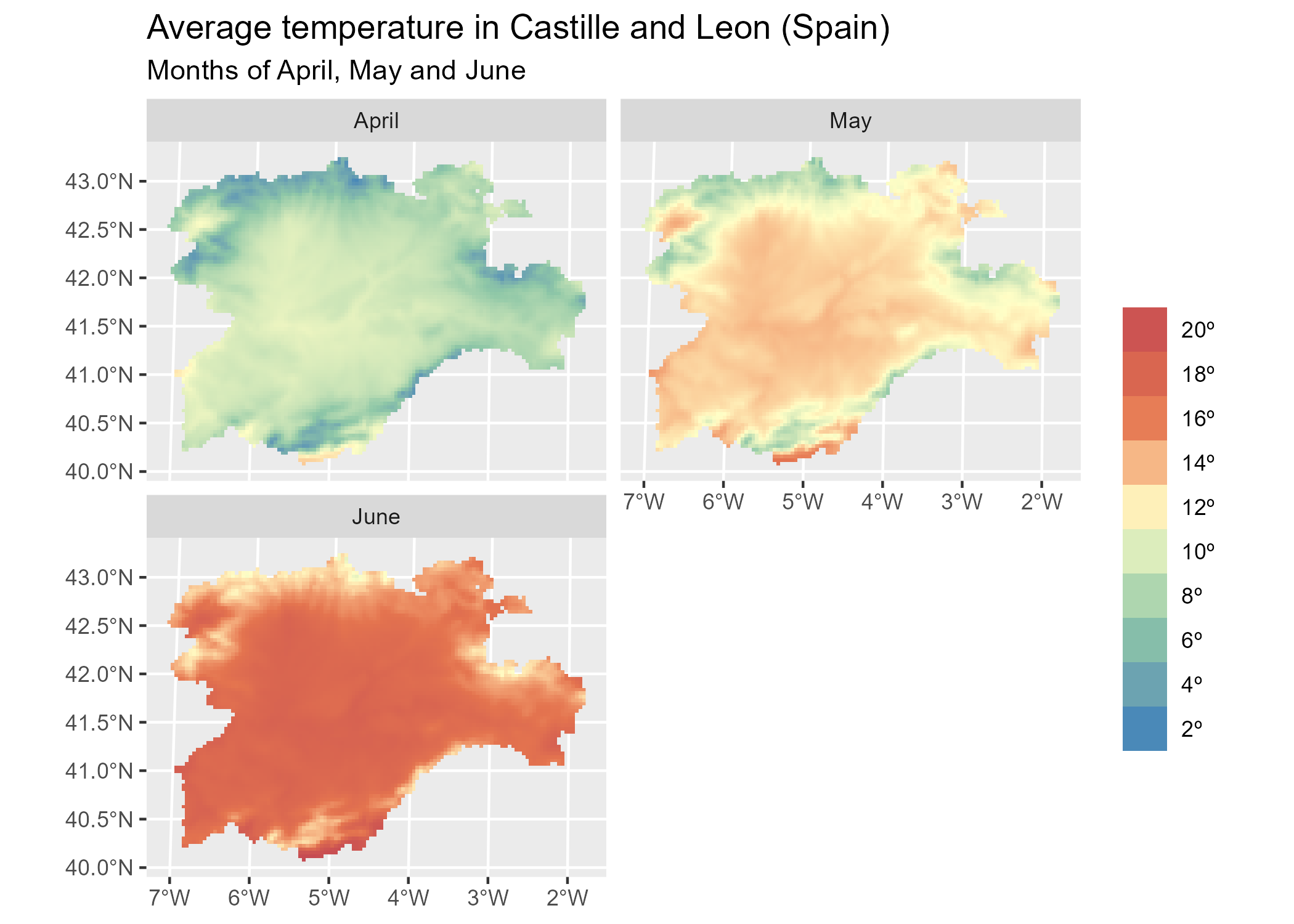``````
# Create maximum differences of two months
variation <- rastertemp %>%
mutate(diff = June - May) %>%
select(variation = diff)

# Add also a overlay of a SpatVector
prov <- vect(system.file("extdata/cyl.gpkg", package = "tidyterra"))

ggplot(prov) +
geom_spatraster(data = variation) +
geom_spatvector(fill = NA) +
scale_fill_whitebox_c(
palette = "deep", direction = -1,
labels = scales::label_number(suffix = "º"),
n.breaks = 5
) +
theme_minimal() +
coord_sf(crs = 25830) +
labs(
fill = "variation",
title = "Variation of temperature in Castille and Leon (Spain)",
subtitle = "Average temperatures in June vs. May"
)``````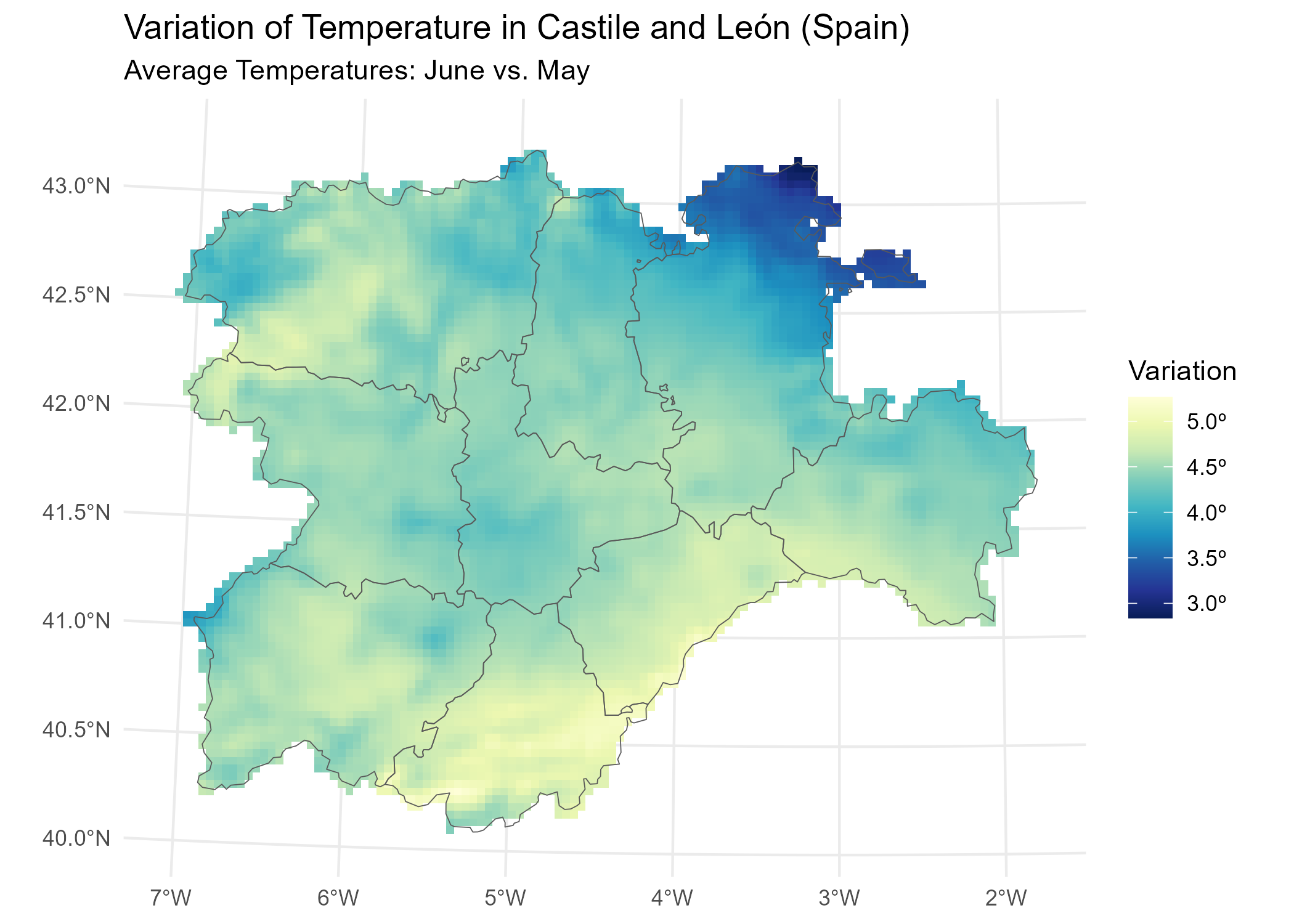{tidyterra} also provide a geom for plotting RGB SpatRaster tiles with {ggplot2}

``````rgb_tile <- rast(system.file("extdata/cyl_tile.tif", package = "tidyterra"))

plot <- ggplot(prov) +
geom_spatraster_rgb(data = rgb_tile) +
geom_spatvector(fill = NA) +
theme_light()

plot``````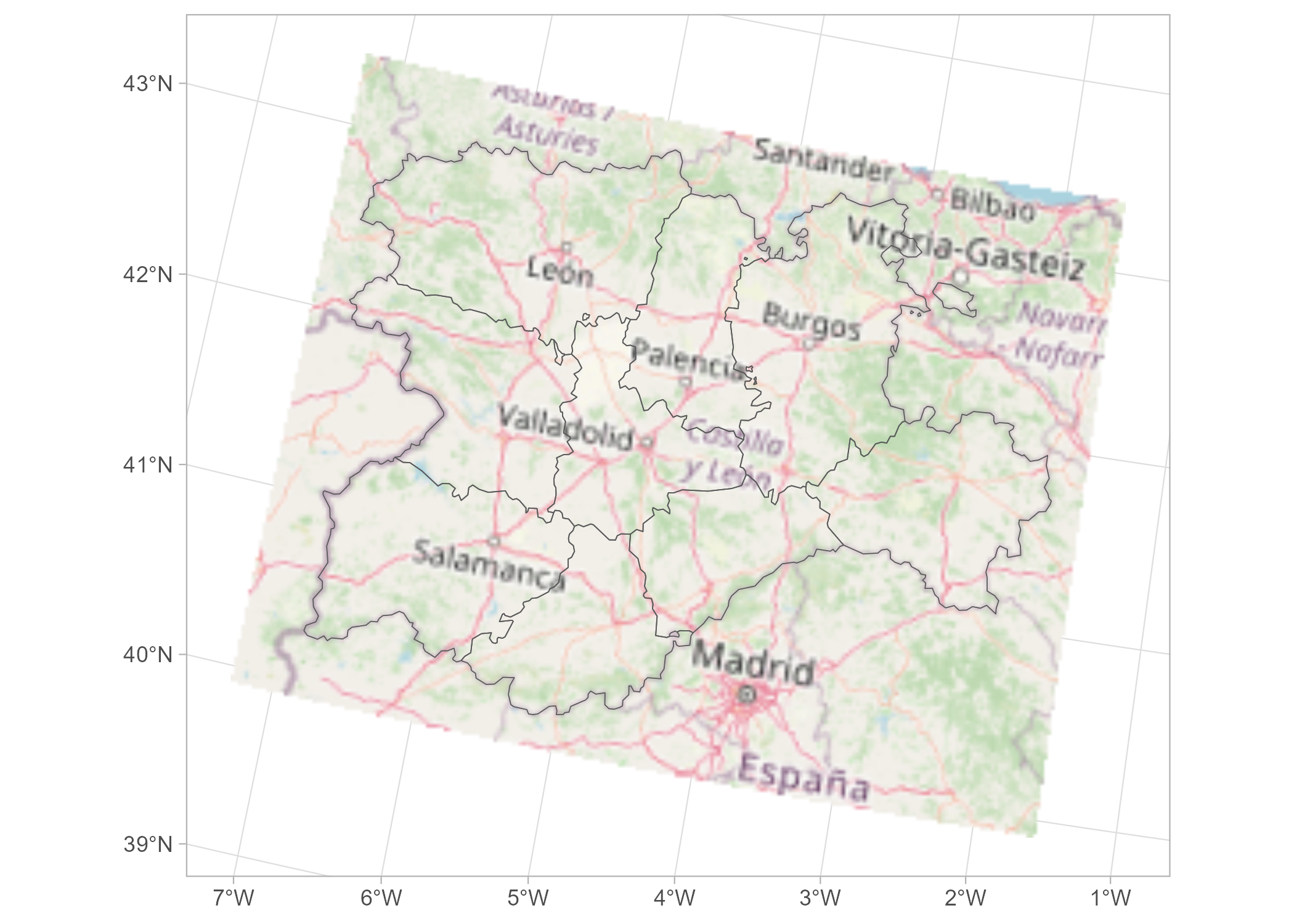``````
# Recognizes coord_sf()
plot +
# Change crs and datum (for relabeling graticules)
coord_sf(crs = 3857, datum = 3857)``````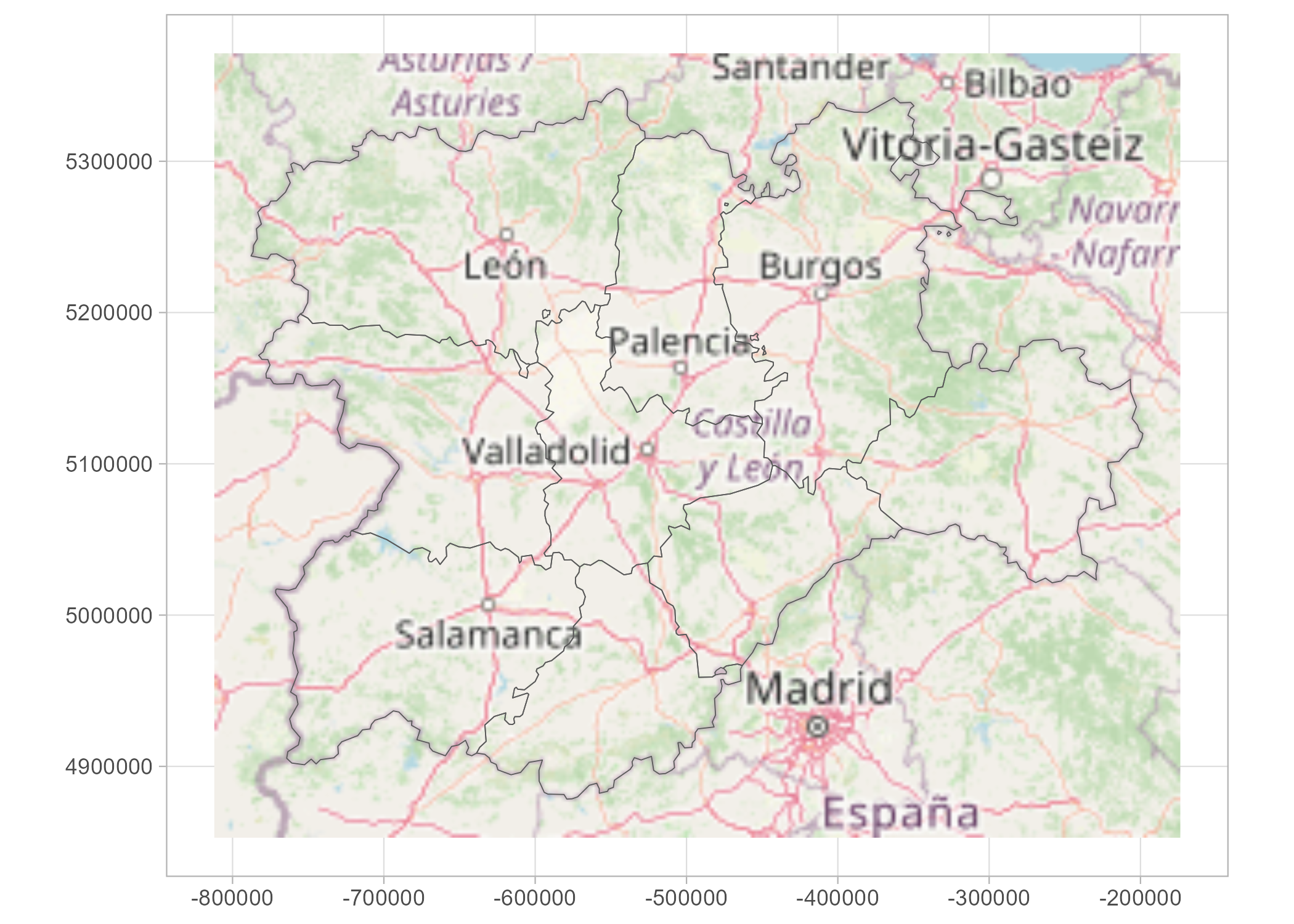{tidyterra} provides specific scales for plotting hypsometric maps with {ggplot2}:

``````asia <- rast(system.file("extdata/asia.tif", package = "tidyterra"))

terra::plot(asia)``````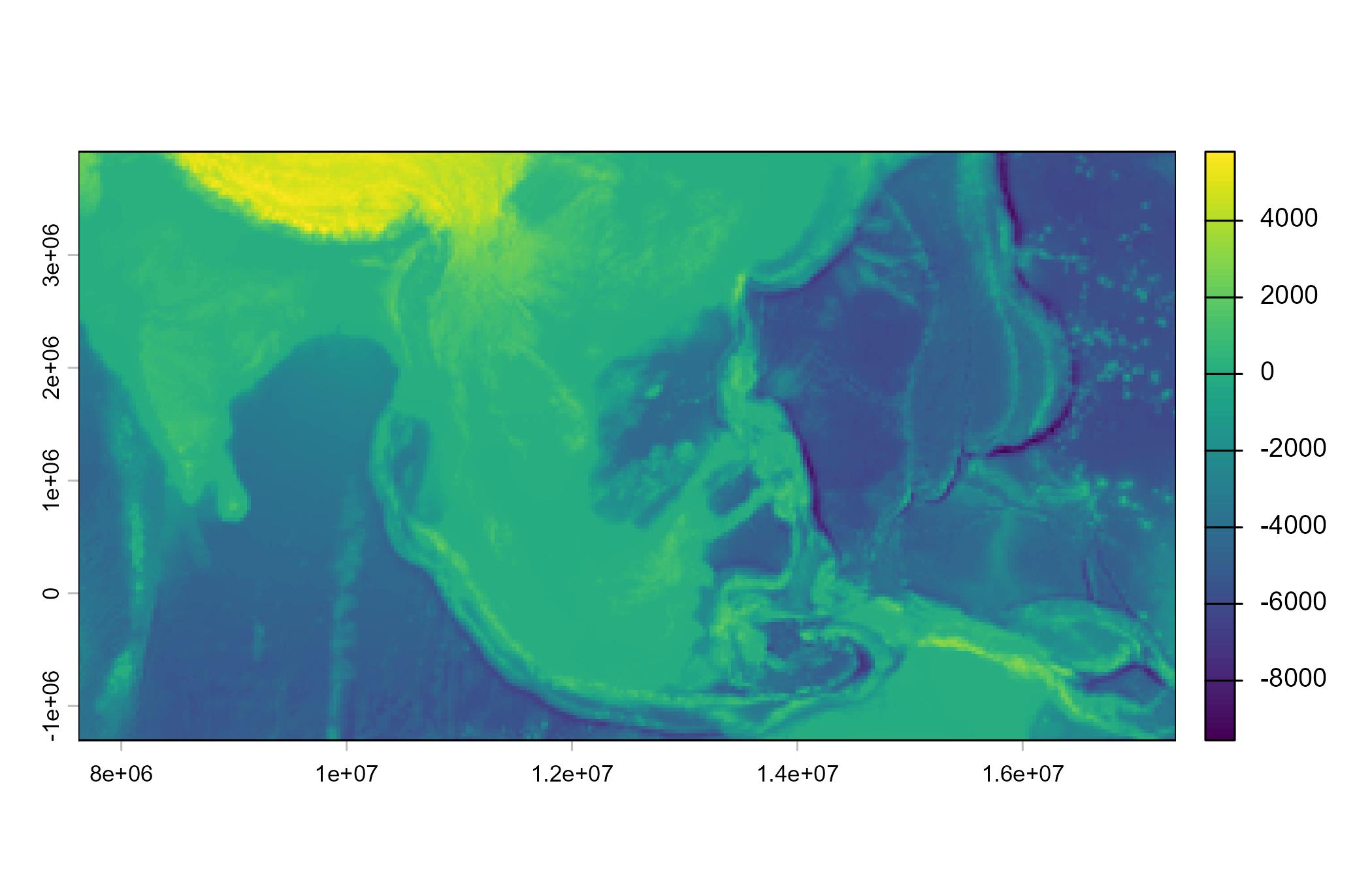``````
ggplot() +
geom_spatraster(data = asia) +
scale_fill_hypso_tint_c(
palette = "gmt_globe",
labels = scales::label_number(),
# Further refinements
breaks = c(-10000, -5000, 0, 2000, 5000, 8000),
guide = guide_colorbar(
direction = "horizontal",
nrow = 1,
title.position = "top",
barwidth = 20,
ticks.colour = "black",
ticks.linewidth = 0.3
)
) +
labs(
fill = "elevation (m)",
title = "Hypsometric map of Asia"
) +
theme(legend.position = "bottom")``````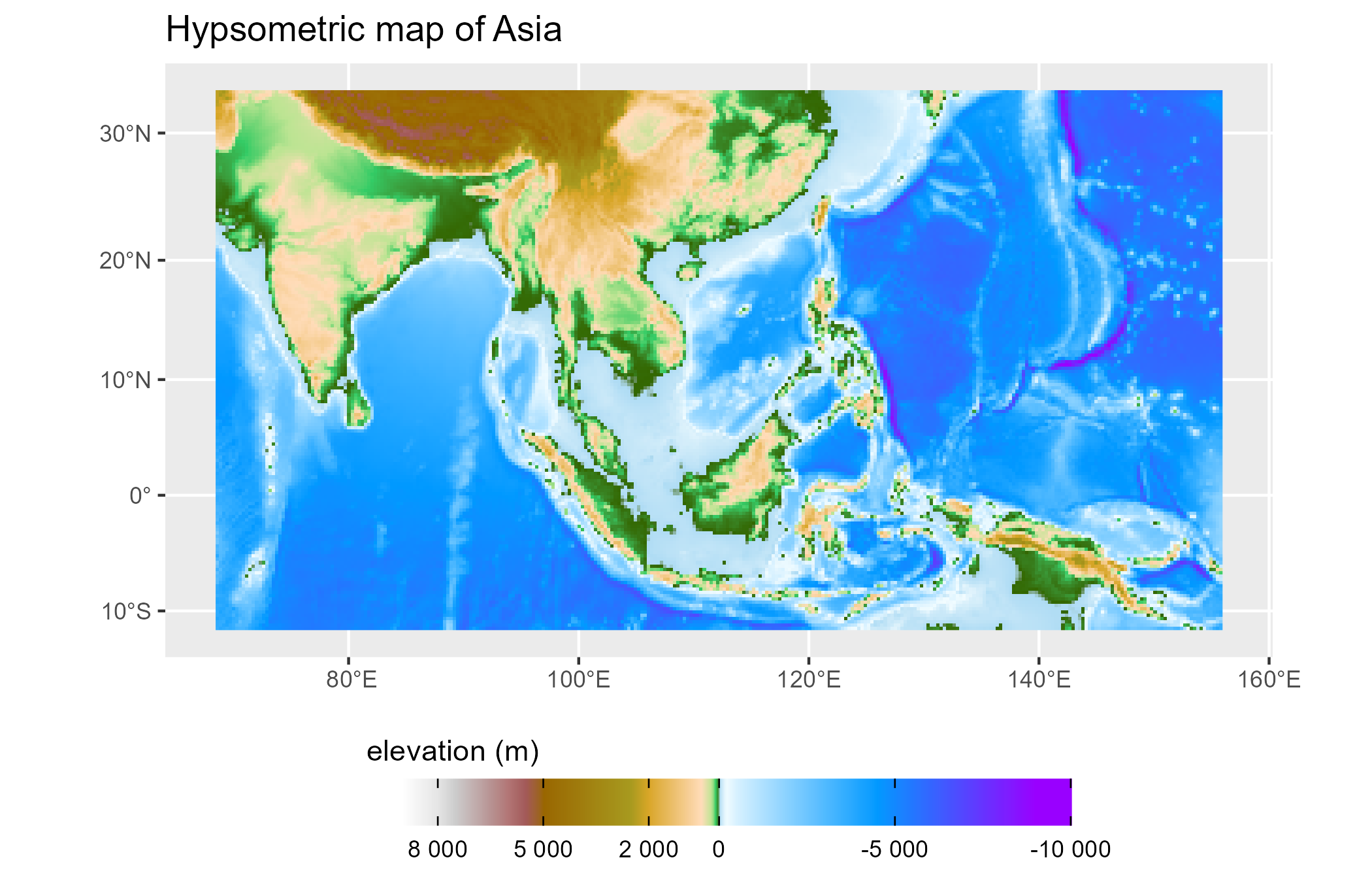### SpatVectors

This is a basic example which shows you how to manipulate and plot SpatVector objects:

``````vect(system.file("ex/lux.shp", package = "terra")) %>%
mutate(pop_dens = POP / AREA) %>%
glimpse() %>%
autoplot(aes(fill = pop_dens)) +
scale_fill_whitebox_c(palette = "pi_y_g") +
labs(
fill = "population per km2",
title = "Population density of Luxembourg",
subtitle = "By canton"
)
#> Geometry type: Polygons
#> Geodetic CRS: lon/lat WGS 84 (EPSG:4326)
#> Extent (x , y) : [5° 44' 38.9045" E - 6° 31' 41.7076" E] , [49° 26' 52.1063" N - 50° 10' 53.8376" N]
#> Rows: 12
#> Columns: 7
#> \$ ID_1     <dbl> 1, 1, 1, 1, 1, 2, 2, 2, 3, 3, 3, 3
#> \$ NAME_1   <chr> "Diekirch", "Diekirch", "Diekirch", "Diekirch", "Diekirch", "…
#> \$ ID_2     <dbl> 1, 2, 3, 4, 5, 6, 7, 12, 8, 9, 10, 11
#> \$ NAME_2   <chr> "Clervaux", "Diekirch", "Redange", "Vianden", "Wiltz", "Echte…
#> \$ AREA     <dbl> 312, 218, 259, 76, 263, 188, 129, 210, 185, 251, 237, 233
#> \$ POP      <int> 18081, 32543, 18664, 5163, 16735, 18899, 22366, 29828, 48187,…
#> \$ pop_dens <dbl> 57.95192, 149.27982, 72.06178, 67.93421, 63.63118, 100.52660,…``````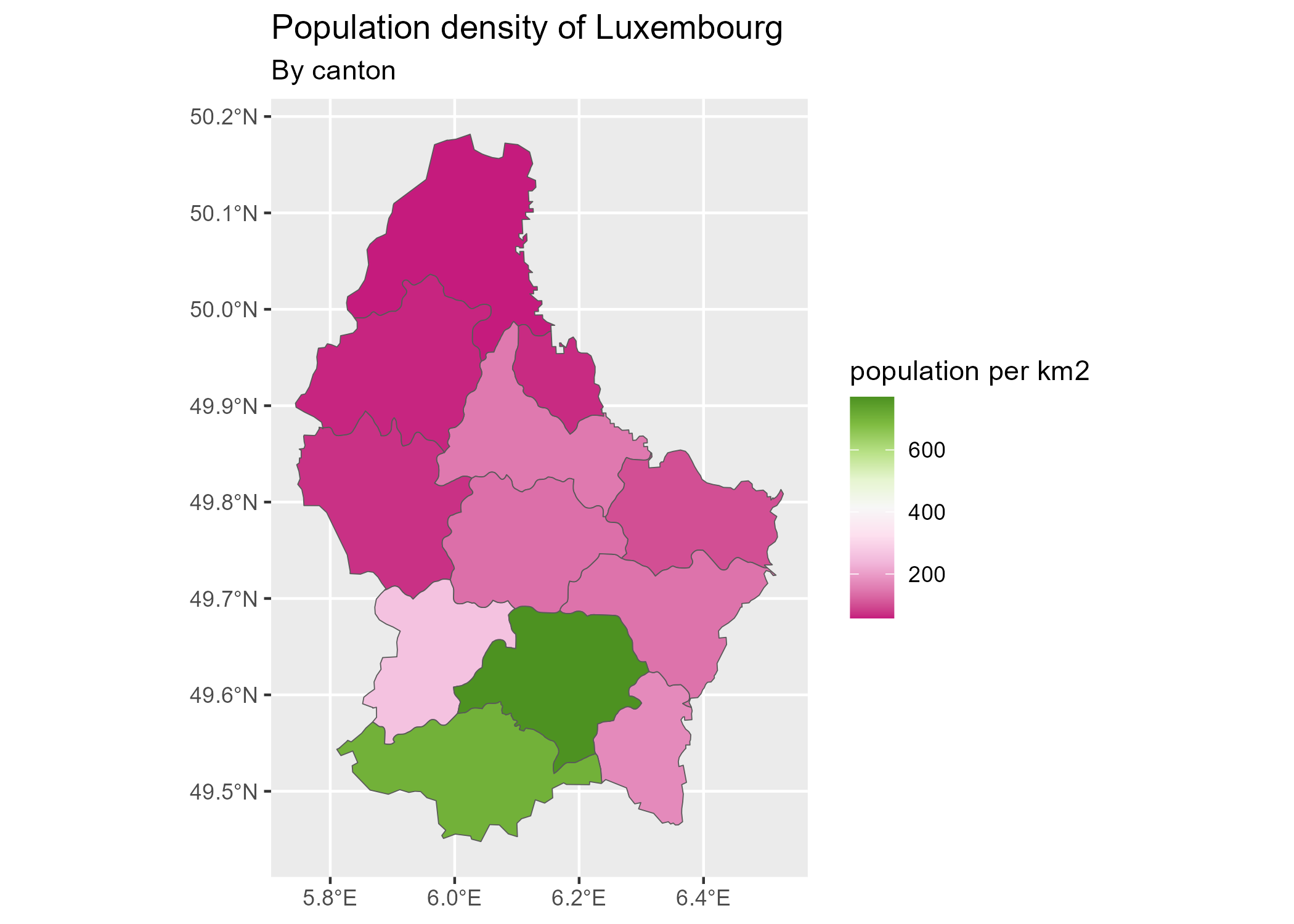{tidyterra} is currently on development mode. Please leave your feedback or open an issue on https://github.com/dieghernan/tidyterra/issues.

## Need help?

Check our FAQs or open a new issue!

You can also ask in Stack Overflow using the tag `tidyterra`.

## Citation

If you use this software, please cite our article in the Journal of Open Source Software:

Hernangómez, D., (2023). Using the tidyverse with terra objects: the tidyterra package. Journal of Open Source Software, 8(91), 5751, https://doi.org/10.21105/joss.05751.

A BibTeX entry for LaTeX users is

``````@Article{R-tidyterra,
title = {Using the {tidyverse} with {terra} objects: the {tidyterra} package},
doi = {10.21105/joss.05751},
author = {Diego Hernangómez},
year = {2023},
url = {https://doi.org/10.21105/joss.05751},
publisher = {The Open Journal},
volume = {8},
number = {91},
pages = {5751},
journal = {Journal of Open Source Software},
issn = {2475-9066},
}``````

## Acknowledgement

{tidyterra} ggplot2 geoms are based on {ggspatial} implementation, by Dewey Dunnington and ggspatial contributors.